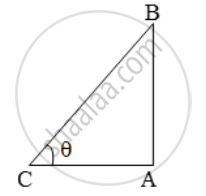# If a vertical pole 6m high has a shadow of length 2 √3 metres, find the angle of elevation of the sun. - Mathematics

Sum

If a vertical pole 6m high has a shadow of length 2 √3 metres, find the angle of elevation of the sun.

#### Solution

Let AB be the vertical pole and AC be its shadow.Let the angle of elevation be θ. Then,

AB = 6 m, AC = 2 √3 m and ∠ACB = θ.

\text{Now, tan }\theta=\frac{AB}{AC}=\frac{6}{2\sqrt{3}}=\sqrt{3}=\text{ tan }60^\text{o}.

∴ θ = 60º.

Concept: Heights and Distances
Is there an error in this question or solution?